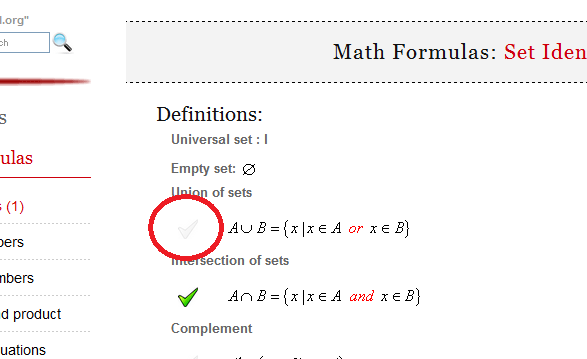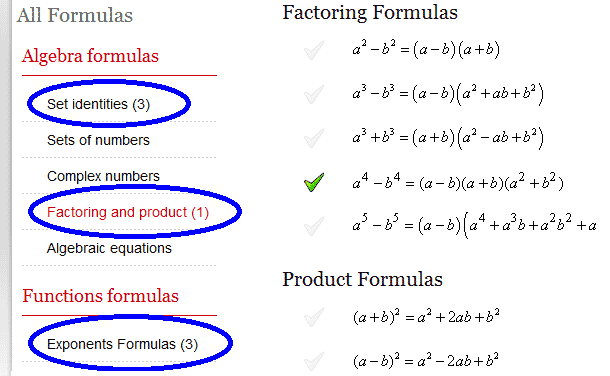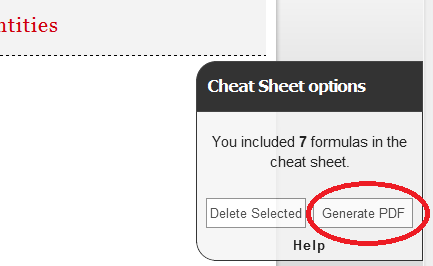Math Calculators, Lessons and Formulas

It is time to solve your math problem

mathportal.org

You included 0 formulas in your custom cheat sheet.

Cheat Sheet options

## How to generate cheat sheet ?

To create cheat sheet first you need to select formulas which you want to include in it. To select formula click at picture next to formula.You can choose formulas from different pages.After you have selected all the formulas which you would like to include in cheat sheet, click the "Generate PDF" button.# Math Formulas: Sets of Numbers

### Definitions:

$\mathbb{N}$ : Natural numbers

$\mathbb{N}_0$ : Whole numbers

$\mathbb{Z}$ : Integers

$\mathbb{Z}^+$ : Positive integers

$\mathbb{Z}^-$ : Negative integers

$\mathbb{Q}$ : Rational numbers

$\mathbb{C}$ : Complex numbers

### Formulas:

Natural numbers (counting numbers )

 $$\mathbb{N} = \left\{ 1, 2, 3, \dots \right\}$$

Whole numbers ( counting numbers with zero )

 $$\mathbb{N}_0 = \left\{0, 1, 2, 3, \dots \right\}$$

Integers ( whole numbers and their opposites and zero )

 $$\mathbb{Z} = \left\{ \dots , -2, -1, 0, 1, 2, \dots \right\}$$
 $$\mathbb{Z}^+ = \mathbb{N} = \left\{ 1, 2, \dots \right\}$$
 $$\mathbb{Z}^- = \left\{ \dots , -3, -2, -1 \right\}$$
 $$\mathbb{Z} = \mathbb{Z}^- \cup { 0 } \cup \mathbb{Z}$$

Irrational numbers: Non repeating and nonterminating integers

Real numbers: Union of rational and irrational numbers

Complex numbers:

 $$\mathbb{C} = \left\{ x+iy ~|~ x \in \mathbb{R} ~~ and ~~ y \in \mathbb{R} \right\}$$
 $$\mathbb{N} \subset \mathbb{N}_0 \subset \mathbb{Z} \subset \mathbb{Q} \subset \mathbb{R} \subset \mathbb{C}$$